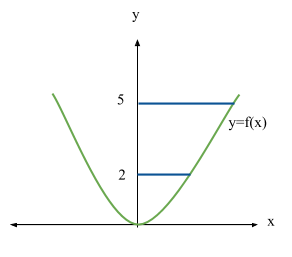# Volume of Solids of Revolution

Contents

### Summary

• Rotating about the x axis, Volume of Revolution:$v\quad =\quad \int _{ a }^{ b }{ \pi { y }^{ 2 } } dx$

• Rotating about the y axis, Volume of Revolution:$v\quad =\quad \int _{ a }^{ b }{ \pi { x }^{ 2 } } dy$

#### Definite Integrals Formula

We know that definite integrals can help us figure out areas underneath the curves.

Recalling the formula for definite integrals from the article ”Integration” :$\int _{ a }^{ b }{ f^{ ' }\left( x \right) } dx\quad =\quad f\left( b \right) \quad -\quad f\left( a \right)$

Similarly, pretty much using the same principle we can work out volumes of rotating solids.

If the part of a curve y = f(x) between the giving limits x = a & x = b is rotated about the x axis or y axis through 360°, then the solid formed is called a ‘Solid of Revolution’.

Such a solid is always symmetrical about the axis of rotation. Examples of such solids are, cone, cylinder and sphere etc.

Consider an element with radius y and height$\Delta x$.Volume of the element$=\quad \pi { y }^{ 2 }\Delta x$

Volume of the entire solid$=\quad \sum _{ a }^{ b }{ \pi { y }^{ 2 }\Delta x }$

Note: The smaller the$\Delta x$,  the approximation is closer to the actual value.

When$\Delta x\quad \rightarrow \quad 0$ :$v\quad =\quad \int _{ a }^{ b }{ \pi { y }^{ 2 } } dx$

Hence, we can deduce that:

The volume of revolution of y = f(x) between x = a & x = b about the axis of x is:$v\quad =\quad \int _{ a }^{ b }{ \pi { y }^{ 2 } } dx$

Let’s look at some examples now.

#### Example 1Q. The region between the curve$y\quad =\quad f\left( x \right) \quad =\quad { x }^{ 2 }$,  the x axis and the line x = 1 and x = 3 is rotated through 360° about the x axis.

Find the volume of revolution which is formed.

Solution:

Using the formula$v\quad =\quad \int _{ a }^{ b }{ \pi { y }^{ 2 } } dx$

Replacing y with${ x }^{ 2 }$,$v\quad =\quad \int _{ 1 }^{ 3 }{ \pi { ({ x }^{ 2 }) }^{ 2 } } dx$$=\quad\pi \int _{ 1 }^{ 3 }{ { x }^{ 4 } } dx$$=\quad \pi { \left[ \frac { { x }^{ 5 } }{ 5 } \right] }_{ 1 }^{ 3 }$$=\quad \frac { \pi }{ 5 } (243\quad -\quad 1)$

Ans:$=\quad \frac { 242\pi }{ 5 }$ cubic units

#### Example 2Q. The region between the curve$y\quad =\quad f\left( x \right) \quad =\quad { x }^{ 2 }$,  the y axis and the line y = 2 and y = 5 is rotated 360° around the y axis.

Find the volume of revolution obtained.

Solution:

Using the formula$v\quad =\quad \int _{ a }^{ b }{ \pi { x }^{ 2 } } dy$

where${ y }^{ 2 }$  is now replaced by${ x }^{ 2 }$

Hence:$v\quad =\quad \int _{ 2 }^{ 5 }{ { \pi y } } dy$  as$y\quad =\quad { x }^{ 2 }$$=\quad\pi \int _{ 2 }^{ 5 }{ { y } } dy$$=\quad \pi { \left[ \frac { { y }^{ 2 } }{ 2 } \right] }_{ 2 }^{ 5 }$$=\quad \pi (\frac { 25 }{ 2 } \quad -\quad \frac { 4 }{ 2 } )$

Ans:$=\quad \frac { 21\pi }{ 2 }$  cubic units

##### Reference
1. http://amsi.org.au/teacher_modules/Cones_Pyramids_and_Spheres.html
2. MEI A Level Further Mathematics Year 2 4th Edition